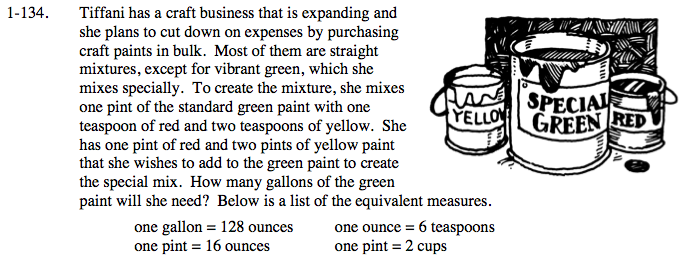### Home > PC > Chapter 1 > Lesson 1.4.1 > Problem1-134

1-134.Find out how many batches of paint one pint of red could make.

$\left(\frac{\text{6 teaspons}}{\text{ounce}}\right)\left(\frac{\text{16 ounces}}{\text{pint}}\right)=96\text{ batches}$
$\frac{1\ \text{gallon\ green}}{8\ \text{batches}}= \frac{x\text{\ number\ of\ gallons\ of\ green}}{96\text{\ batches}}$